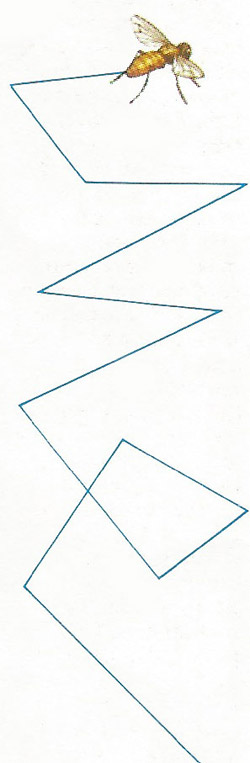A# random walkA fly has an instinctive system of evading predators using an aerobatic pattern. As game theory recommends, it keeps making random alterations of course at random times. Its course is then safely unpredictable, even to itself.

A random walk is a process in which the position of a particle changes by discrete steps of fixed length, and the direction of each step is chosen at random. Random walks have interesting mathematical properties that vary greatly depending on the number of dimensions in which the walk takes place and whether it is confined to a lattice.

For a random walk in one dimension there are only two directions to choose from. Imagine a drunken person wandering on the number line who starts at 0, and then moves left or right (+/–1) with probability 1/2. The probability that the walker will eventually return to his starting point is 1; in other words, it is certain to happen. The same is true for a random walk in the plane, moving on the integer lattice points, with probability 1/4 in each of the coordinate directions: the probability of ending up back at the starting point is 1. However, the situation changes in three dimensions. Suppose a drunken fly moves randomly from one point to another in a three-dimensional lattice with a probability of 1 in 6 of arriving at any of the six adjacent lattice points on each move. No matter how long the fly roams, it has only a 0.34054... probability of ever getting back to where it started.

Probability theorists say that random walks on the line and plane are recurrent, whereas random walks in three dimensions or more are transient. Effectively, this is because there is so much more "space" in three or more dimensions. The numbers giving the probability of eventually returning to the starting point are known as random walk constants. The random thermal perturbations in a liquid are responsible for a random walk phenomenon known as Brownian motion, and the collisions of molecules in a gas are a random walk responsible for diffusion.